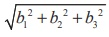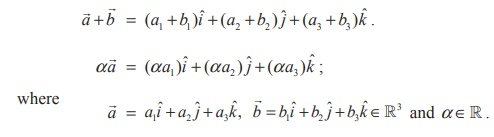Home | | Maths 12th Std | Geometric introduction to vectors

# Geometric introduction to vectors

Mathematics : Geometric introduction to vectors

Geometric introduction to vectors

A vectoris represented as a directed straight line  segment in a 3-dimensional space R3 , with an  initial  point A  =  (a1, a2, a3) R3 and an end point  B  = (b1, b2, b3 ) R3 , and  it is denoted by.  The length of the line segment AB is the magnitude of the vectorand the direction from A to B is the direction of the vector. Hereafter, a vector will be interchangeably denoted  byor. Two vectorsandin R3 are said to be equal if and only if the length AB is equal to the length CD and the direction from A to B is parallel to the direction from C to D . Ifandare equal, we write, andis called a translate of.

It is easy to observe that every vectorcan be translated to anywhere in R3 , equal to a vector with initial point U R3 and end point V R 3 such that. In particular, if O is the origin of  R3 , then a point P R3 can be found such that. The vectoris called the position vector of the point P . Moreover, we observe that given any vector, there exists a unique point P R3 such that the position vectorof P is equal to. A vectoris said to be the zero vector if the initial point A is the same as the end point B . We use the standard notations iˆ, ˆj, kˆ  andto denote the position vectors of the points (1, 0, 0),(0,1, 0),(0, 0,1), and (0, 0, 0), respectively. For a given point (a1, a2, a3) R3, aiˆ + a ˆj + akˆ is called the position vector of the point (a1, a2, a3) which is the directed straight line segment with initial point (0, 0, 0) and end point (a1 , a2 , a3 ) . All real numbers are called scalars.

Given a vector, the length of the vector |is calculated bywhere A is (a1 , a2 , a3 ) and B is (b1 , b2 , b3 ). In particular, if a vector is the position vectorof (b1 , b2 , b3 ), then its length isA vector having length 1 is called a unit vector. We the notation uˆ , for a unit vector. Note that iˆ, ˆj , and kˆ  use are unit vectors andis the unique vector with length 0 . The direction ofis specified according to the context.

The addition and scalar multiplication on vectors in 3-dimensional space are defined byTo see the geometric interpretation of, letand, denote the position vectors of A = (a1 , a2 , a3 ) and B = (b1 , b2 , b3 ) , respectively. Translate the position vectorto the vector with initial point as A and end point as C = (c1 , c2 , c3 ) , for a suitable (c1 , c2 , c3 ) R3. See the  Fig (6.2). Then, the position vectorof the point (c1 , c2 , c3 ) is equal to.

The vector αis another vector parallel toand its length is magnified (if α > 1) or contracted  (if 0 < α < 1) . If α < 0 , then αis a vector whose magnitude is | α | times that ofand direction opposite to that of. In particular, if α = −1, then α= −is the vector with same length and direction opposite to that of. See Fig. 6.3Study Material, Lecturing Notes, Assignment, Reference, Wiki description explanation, brief detail
12th Mathematics : UNIT 6 : Applications of Vector Algebra : Geometric introduction to vectors |